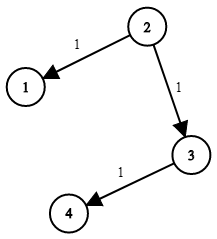# LeetCode 743 - 网络延迟时间

8/4/2021 LeetCode

## # 题目描述1 <= k <= n <= 100
1 <= times.length <= 6000
times[i].length == 3
1 <= ui, vi <= n
ui != vi
0 <= wi <= 100

## # Python题解

### # 方法一：Dijkstra 算法

Dijkstra 算法

    def networkDelayTime(self, times, n, k):
"""
:type times: List[List[int]]
:type n: int
:type k: int
:rtype: int
"""
# 邻接矩阵存储图，此处存储距离信息
g = [[float('inf')] * n for _ in range(n)]
# 从邻接矩阵更新权重，对下标进行了减一处理
for start, end, time in times:
g[start - 1][end - 1] = time
# 目标节点到其他节点的最短距离列表信息
distance = [float('inf')] * n
# 起点距离设置为0
distance[k - 1] = 0
# 用于标记已被经过的节点
used = [False] * n
# 遍历所有节点
for _ in range(n):
# 第一次不用比较距离用到的参数
x = -1
# 获取未被标记的最近节点
for index, false in enumerate(used):
if not false and (x == -1 or distance[index] < distance[x]):
x = index
# 标记节点
used[x] = True
# 更新最短路径距离
for index, time in enumerate(g[x]):
distance[index] = min(distance[index], distance[x] + time)
# 要求所有节点接收，所以返回最短距离的最大值
ans = max(distance)
# 如果存在inf则代表有节点不可达，此时返回-1
return ans if ans < float('inf') else -1

1
2
3
4
5
6
7
8
9
10
11
12
13
14
15
16
17
18
19
20
21
22
23
24
25
26
27
28
29
30
31
32
33
34
35